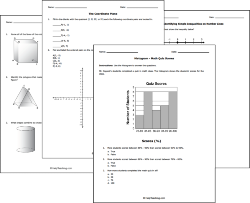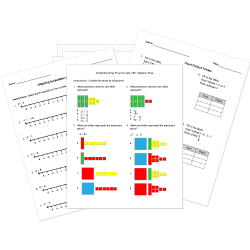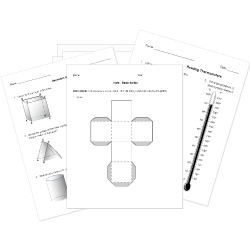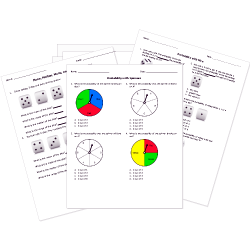Tweet# Printable Middle School Math WorksheetsAssess student understanding of pre-algebra and geometry concepts with these math worksheets for middle school students.

Worksheets labeled with are accessible to Help Teaching Pro subscribers only. Become a Pro Subscriber to access hundreds of standards aligned worksheets.

Don't see a middle school math worksheet you need? Use our Test Maker™ to create your own printable that fits your needs. Browse middle school math questions or use our advanced search to find existing questions while filtering by grade levels and keywords.

Looking for a way to create math questions and worksheets with properly formatted equations? Use our math equation editor. You can add equations to the question text and to multiple choice math questions.

## Middle School Number Systems & AlgebraUse these Number System, Pre-Algebra, and Algebra tests to gauge student comprehension of middle school math topics including: expressions and equations, inequalities featuring number line graphics, functions, exponents, and radicals.

## Middle School GeometryInvestigate lines, angles, circles, triangles, polygons, perimeter, volume, and the coordinate plane, among other topics with these geometry worksheets.

### Statistics and ProbabilityDevelop student understanding of statistical thinking and probability concepts with these worksheets designed for students in grades 6 – 8.